﻿

### Hall Graph of a Finite Group

#### Abstract

The Hall graph of a finite group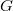$G$ is a simple graph whose vertex set is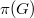$\pi(G)$, the set of all prime divisors of its order, and two distinct primes$p$ and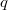$q$ are joined by an edge if$G$ has at least one Hall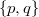$\{p, q\}$-subgroup. For all primes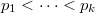$p_1<\cdots of$\pi(G)$, we call the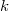$k$-tuple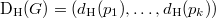${\rm D}_{\rm H}(G)=(d_{\rm H}(p_1), \ldots, d_{\rm H}(p_k))$, the degree pattern of Hall graph of$G$, where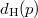$d_{\rm H}(p)$ signifies the degree of vertex$p$. This paper provides some properties of Hall graph. It also gives a characterization for some finite simple groups via order and degree pattern of Hall graph.

DOI Code: 10.1285/i15900932v39n2p25

Keywords: Hall graph; degree pattern of Hall graph; Hall subgroup; simple group

Full Text: PDF